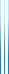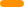# PublicationsPapers

## Efficient voting via the top-$k$ elicitation scheme: a probabilistic approach

Yuval Filmus and Joel Oren
EC 2014

Many voting rules require the voters to give a complete preference order over the candidates. This is cumbersome, leading to the notion of top-$k$ voting, in which the voters only give the length-$k$ prefixes of their rankings. The question that we ask in this paper is: given a voting rule, for what value of $k$ is it possible to predict the overall winner given only the length-$k$ prefixes, with high probability, given enough voters?

We first consider the case of an impartial culture, in which the voters choose their preference profiles uniformly at random over all permutations. For positional scoring rules (like Borda) we give a nearly-tight threshold theorem for $k$. We also prove a strong, though non-optimal, lower bound for Copeland.

When the preference profiles are drawn from a biased distribution, such as the Mallows distribution, we show that the candidate toward which the distribution is biased wins the elections, for both positional scoring rules and Copeland, with high probability.

Finally, we consider adversarially-chosen preference distributions. We show that for positional scoring rules with geometrically decaying scores, $k = O(\log n)$ suffices to predict the winner with high probability.

## BibTeX

@inproceedings{FO2014,
author = {Yuval Filmus and Joel Oren},
title = {Efficient voting via the top-$k$ elicitation scheme: a
probabilistic approach},
booktitle = {Electronic Commerce (EC)},
year = {2014},
pages = {295--312}
}copy to clipboard